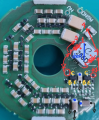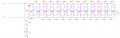# Developing Voltage Multiplier. So, I'm tracking a component that I actually used.

Status
Not open for further replies.

#### Mars Park

Joined Feb 20, 2022
35
I'm developing Voltage Multiplier. So, I'm tracking a component that I actually used. And this picture is the one.First, please check the form of LPD6245. After passing through pins 2 and 4, two signals are input as voltage multipliers. Before the two signals, there is one BJT and one integrator for each signal, but I don't think this is important. (Because, what i want to know is the role of LPD6245) And then, pins 1 and 3 are connected to each other and are grounded through three capacitors. (The blue points) Everything had been checked several times with a multimeter, so I'm sure about this form. The circuit drawn in Orcad is as follows.The coupled coils are LPD6245.

There's something special. The Voltage Multiplier form is unfamiliar. In drawing as followed, The circuit on the top part is a typical Voltage Multiplier seen on the Internet, and the circuit below is in the form of Voltage Multiplier of the part I am tracking. (The diode direction, the position of the GND is inverted, and the direction in which the voltage accumulates is different.)This is all I know. I want to know what LPD6245 works ,, Please give me a lot of advice. Thank you.

Last edited by a moderator: